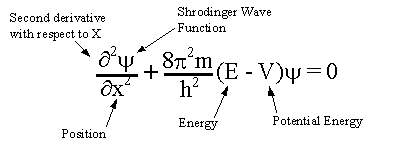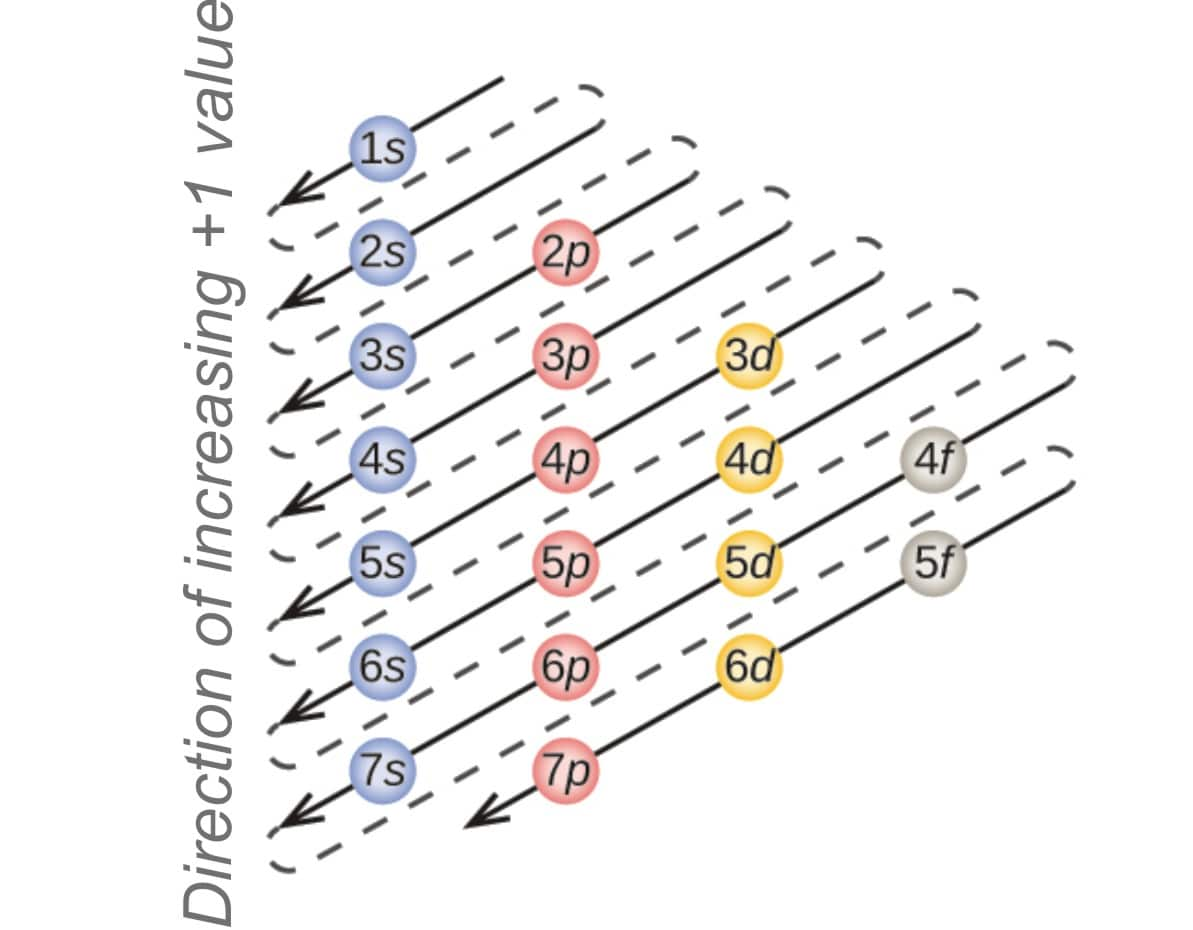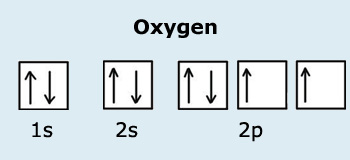# Aufbau Principle, Paulis Exclusion Principle and Hands Rule

## What is Aufbau Principle, Paulis Exclusion Principle and Hands Rule

INTRODUCTION:

We are all aware of the fact that matter is made up of small particles called atoms which further comprise of electrons, protons, neutrons, and nucleus. But in ancient times, it was believed that atoms were the smallest particles and could not be further subdivided. In 1803, John Dalton formulated the solid sphere model. This model was made up of very minute particles which were invisible, these particles were atoms and they were alike. He also put forward the law of multiple proportions and the law of definite composition through this model. Dalton's model consisted of many flaws and failed at explaining many features of atoms.

In 1906, Ernest Rutherford came with the nuclear model of the atom. He conducted the gold foil experiment and proposed the theory that there exists a concentrated mass of positive charge at the center of an atom which is known as a nucleus. Since the negatively charged particles were deflected by the concentrated mass, it must be positively charged. Also, the rest of the model is basically empty space. The main drawback of Rutherford's model was that it could also not explain the stability of an atom. According to his model, the electrons revolving around the nucleus should emit energy and hence would gradually slow down and following a spiral path, would collapse into the nucleus and hence the atom would not be stable and will not exist.

In 1915, Niels Bohr was the first to put forward the theory that the periodicity of elements in the periodic table was due to their electronic configuration. He proposed that electrons revolve around the nucleus of an atom in an orbit at different energy levels. His model is referred to as the planetary model. Bohr’s model could not account for the elements which contained more than one electron. His model was not in accordance with the Heisenberg’s Uncertainty Principle.

All of the above-proposed models were based on the hypothesis that matter had particle nature, that is, it behaves like a particle. Then came the wave mechanics. In 1921, Louis de Broglie stated that if waves have particle nature then matter should also possess wave nature. His theory proved to be correct. In 1925, the Bohr model was replaced by Wolfgang Pauli's model. Wolfgang Pauli's model was based on quantum mechanics. Since then many changes were instilled in the model and it was improved to bring about the modern model by Erwin Schrodinger.

ELECTRONIC CONFIGURATION:

The electronic configuration of an atom provides us with information regarding the different properties of the element like the energy and shape of the orbitals which is responsible for various properties. It helps us understand the position and behavior of the elements contained in the periodic table. It also gives an insight into the various chemical bonds that are formed.

Schrodinger formulated an equation which is known as the Schrodinger equation:When this Schrodinger’s equation for the Bohr’s model is solved then that gives us the three principal numbers known as the quantum numbers. These numbers are; the principal quantum number (n), the angular momentum quantum number of the orbit or the azimuthal quantum number (l), and the magnetic quantum number (ml). The fourth quantum number known as the spin multiplicity or ms is not a solution of the Schrodinger’ equation for the Bohr’s model.

QUANTUM NUMBERS:

In a quantum system, the quantum numbers represent the algebraic values of the conserved quantities. When the Schrodinger equation is solved for the Bohr’s Hydrogen model then the resultant solutions depict the quantum numbers. They describe the energy levels, spin numbers, angular momentum etc of the electrons occupying the orbits. At least one quantum number exists for any quantized system. The four quantum numbers which can provide information about the electrons present in any orbit are:

1) PRINCIPAL QUANTUM NUMBER (n): The principal quantum number provides information regarding the position of the electron, as in the orbit in which it is located. It always takes discrete values, that is, they are always integer values. As the value of n increases then the number of shells also increases. This also means that as the value of n increases then the electrons are residing at a higher energy level.

According to the Schrodinger wave equation, the energy of the electrons in a hydrogen atom is given by:
En= E1/n2

This implies that, En= -13.6 eV/ n2

Where n=1,2,3….
n can take only positive values. And should be an integer.

Now according to Bohr’s model, the angular momentum L is given by;
L= nh/2π

Where n=1,2,3… and h is Plank’s constant

The shells of the elements are denoted on the basis of the principal quantum number as follows
K (n = 1), L (n = 2), M (n = 3)

n is related to the radial quantum number, nr as:

n= nr+l+1

where l is the azimuthal quantum number.

2) AZIMUTHAL QUANTUM NUMBER(l):

The azimuthal quantum numbers are responsible for providing information about the shape of the orbitals. It also determines the orbital angular momentum of the electrons.

The azimuthal numbers 0,1,2,3,4….represent the subshells s,p,d,f and so on, respectively.

l=0 and s subshell is spherical in shape.

l=1, p subshell is three dumbbell shaped orbitals which are oriented along the polarities. That is one lobe of the dumbbell on each pole of the x,y,z- axes.

For l=3, f subshell the shape is quite unique.

One angular momentum state can contain a maximum of 2(2l + 1) electrons.

This arises due to the dependence of the third quantum number, that being the magnetic quantum number(ml) on the azimuthal quantum number as it can take values ranging from -l to +l.

3) MAGNETIC QUANTUM NUMBER (ml):

The magnetic quantum number is used to differentiate between the orbitals which are present in a particular subshell. It can obtain values ranging from -l to +l.

4) SPIN QUANTUM NUMBER (ms):
The spin quantum number denotes the orientation of electrons present in a subshell.

The electrons while filling up the orbitals must follow three specific set of rules. These are:

1) AUFBAU’S PRINCIPLE:

The word Aufbau is derived from the German word Aufbauprinzip which means “principle of building up”. The Aufbau principle states that the orbitals of the higher energy levels could be filled only when the orbitals of the lower levels are filled. Hence the filling up of the electrons take place in increasing order in terms of energy levels of the orbitals.

The correct order of filling up of the orbitals is:

1s 2s 2p 3s 3p 4s 3d 4p 5s 4d 5p 6s 4f 5d 6p 7s 5f 6d 7p

This is based upon the Madelung rule which is the rule of (n+l), meaning the summation of the principal quantum number and the azimuthal quantum number. This is directly proportional to the energy level of the orbit. So, if the value of n+l is low then the orbital energy is also low, if it is high then the orbital energy is also high.2) HUND’S RULE:

Hund’s rule or the Hund’s rule of maximum multiplicity states that the electrons will first singly fill the two or more available orbitals of the same energy levels (degenerate orbitals) at a time.

The second thing to be kept in mind is that after the electrons singly fill the degenerate orbitals, they should be parallel while doubly filling the orbitals. Or, all of the electrons while singly occupying the orbitals should possess the same spin in order to provide maximum value of total spin.

Let’s take oxygen as an example;

The atomic number of oxygen is 8. The electronic configuration of oxygen is: 1s2 2s2 2p4Here the electrons first occupy the vacant orbitals singly and then the electrons which are left doubly occupy the orbitals.

This happens because of like charges repel each other. Meaning that electrons carry a negative charge and they repel each other and so in order to minimize this repulsion the process of occupying other orbits rather than sharing and pairing up, is preferred. Furthermore, the shielding effect of the nucleus is less on the single present electrons.

3) PAULI EXCLUSION PRINCIPLE:

Pauli exclusion principle was postulated by Wolfgang Pauli. The principle states that in a poly-electron system, two electrons cannot have the same value of the four quantum numbers. This means that, if two electrons are occupying the same orbital that is their principal quantum number is same and they even have the same values for azimuthal quantum numbers and the magnetic quantum number then their spin number must be different that is, ½ and -½.